Courses
Courses for Kids
Free study material
Free LIVE classes
More

# HCF By Long Division MethodLIVE
Join Vedantu’s FREE Mastercalss

As we all know, the Highest Common Factor (HCF) as the name itself says, it's the method of finding the highest common factors of two or more than two numbers. It's the highest common number that can divide the given two or more two positive numbers equally.

There are different methods through which we can find out the HCF of given numbers. Of Course, it can be used to find the HCF of small numbers but when it comes to large numbers, then the most suitable method is the Long Division Method. Come on, let us understand HCF by the long division method step by step with a few examples along the way.

## What is H.C.F?

HCF stands for Highest Common Factor. HCF of 2 numbers is the highest factor that can divide the two numbers easily. The highest common factor (HCF) can be evaluated for 2 or more than 2 numbers.  It is the greatest divisor for any two or more numbers that can equally or completely divide the given numbers.

For Example, the HCF of 60 and 75 is 15 because 15 is the largest number which can divide both 60 and 75 exactly.

## Methods for Finding H.C.F

HCF of two or more number can be found by using two methods:

• By prime factorization method

• By division method

## Steps of HCF by Long Division Method

Step 1: Divide the larger number by the smaller number.

HCF of 18 and 30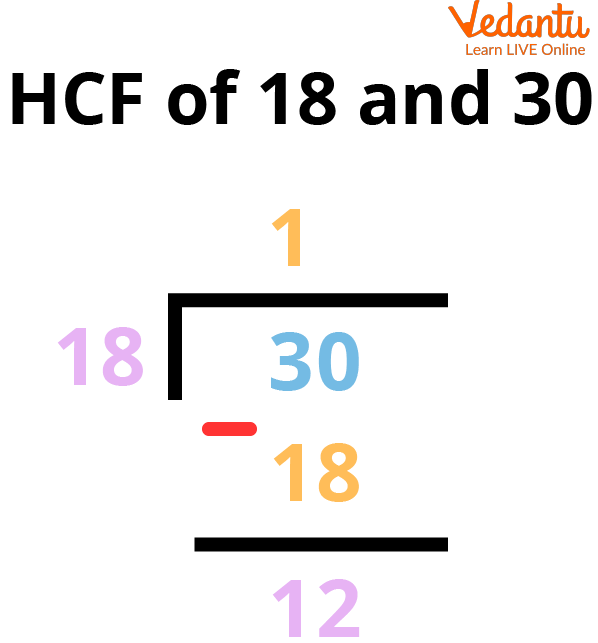The Long Division Method

Step 2: The remainder becomes the divisor and the divisor becomes the dividend. Therefore, divide the first divisor by the first remainder.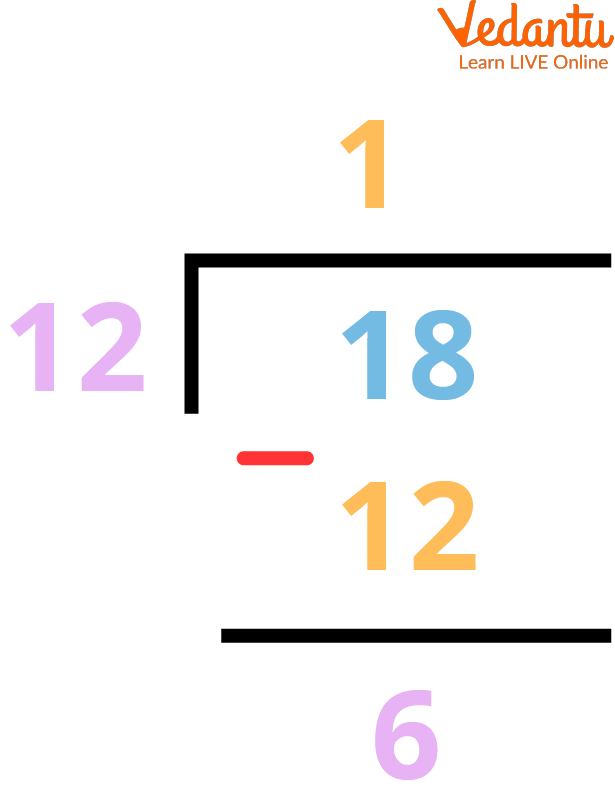The Long Division Method

Step 3: If the remainder is not 0, then again the remainder becomes the divisor and the divisor becomes the dividend. Therefore, divide the second divisor by the second remainder.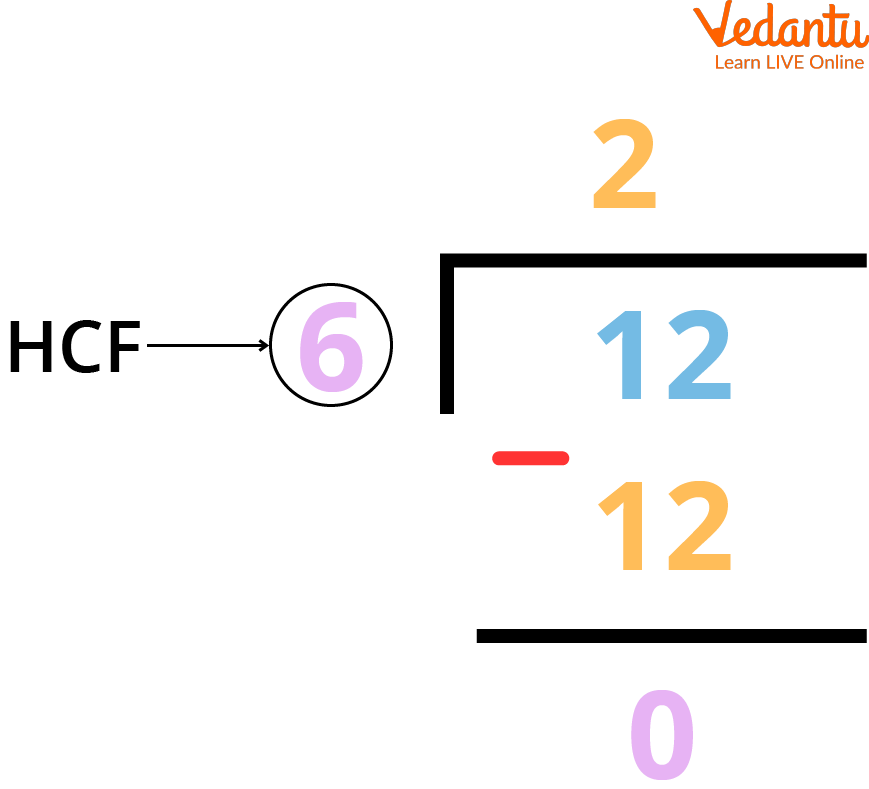The Long Division Method

Step 4: The divisor which does not leave a remainder is the HCF of the two numbers and thus, the last divisor becomes the HCF of the given two numbers.

Let us try to understand the long division method to find the HCF of given numbers with examples.

## Solved Examples

Example 1:Find the HCF of 64 and 144 using the long division method

Step 1: Dividing the larger given number with the smaller given number.  So, 144 becomes the dividend and 64 as the divisor.Long Division Method

Step 2: The remainder becomes the divisor and the divisor becomes the dividend.

Step 3: Therefore, we need to divide 64 (dividend) by 16 (divisor).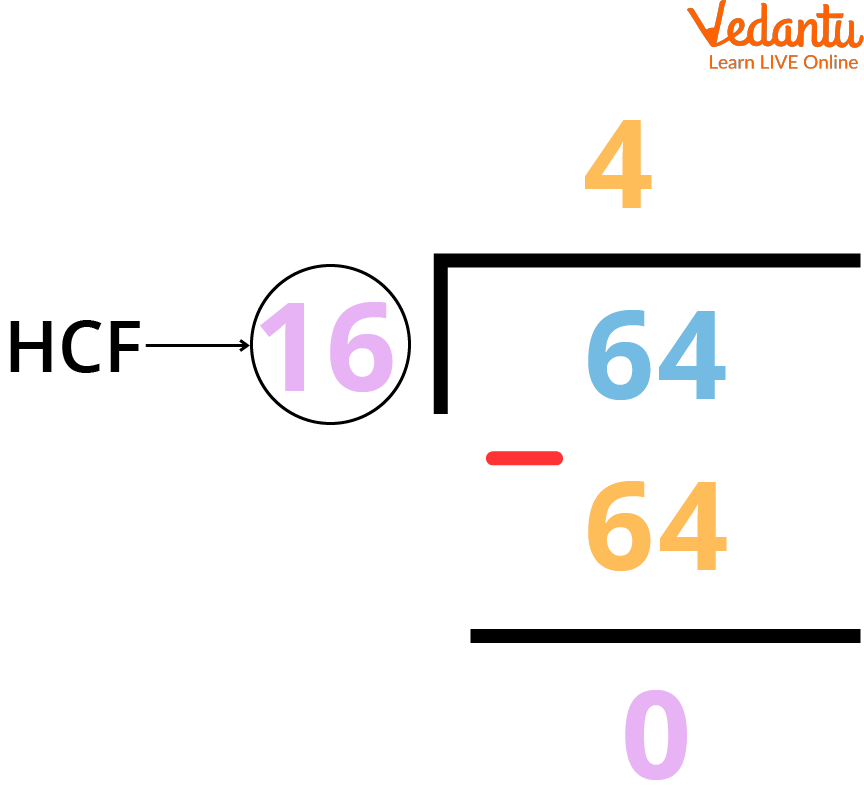Long Division Method

Once the remainder becomes 0, the divisor of that particular division becomes the HCF.

Therefore, HCF of (64, 144) = 16

Example 2: Find the HCF of  700 and 300 using the long division method.

Step 1: Simply divide the larger given number by the smaller given number.  In this case, 300 is the divisor and 700 is the dividend.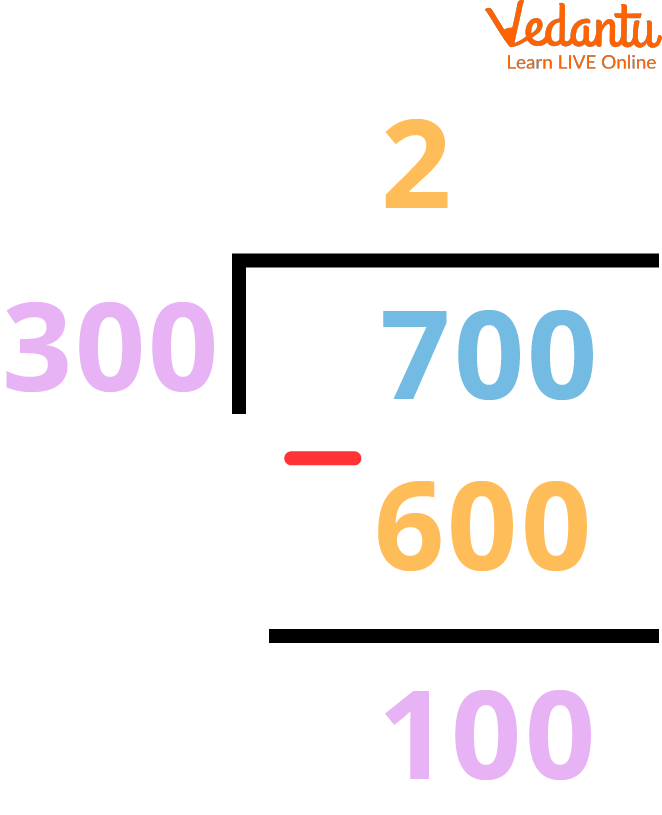Long Division Method

Step 2: The remainder that we got is 100 therefore the remainder that is 100 becomes the divisor and 300 which was the divisor earlier becomes the dividend.Long Division Method

Step 3: Now, we simply divide the second divisor with the second remainder. Follow the image down below for a better understanding.

=> HCF of 700 and 300 is 100

## Practice questions

1. Find the HCF of 30 and 45

Ans: 15

2. Find the HCF of 162 and 180

Ans: 18

3. Find the HCF of 270 and 9

Ans: 9

4. Find the HCF of 78 and 98

Ans: 2

5. Find the HCF of 702 and 405

Ans: 27

## Summary

This article has tried to explain the detailed steps of how to find HCF by using the long Division method for 2 positive given numbers. To find the HCF by division method, the first step is to divide the larger number by the smaller number and then the remainder becomes the divisor and divide the smaller number until the remainder is zero. In this article, you have learn about all other different topics related to HCF.

Last updated date: 21st Sep 2023
Total views: 110.1k
Views today: 3.10k

## FAQs on HCF By Long Division Method

1. What are the other names of H.C.F?

HCF is also known as Greatest Common Measure (GCM) and Greatest Common Divisor (GCD). The HCF or GCD of two numbers is the number which can divide the given two or more than two positive numbers equally without any remainder.  The HCF or GCD measures how many of the numbers can be divided without a remainder by other numbers.  It's important that the remainder is always 0 then only the last divisor can be considered as the HCF in the long division method.

2. Why is HCF calculated and what is its importance?

Although HCF is mainly used in mathematical problems to split things into smaller sections but It can also be used to arrange something into rows and groups for distribution. It is/can be applied in our daily lives as well.  It can be used in real-time scenarios such as buying return gifts for the kids, donating food equally to the orphans, interior decor requirements, etc.

Apart from the long division method, there are several methods through which we can calculate HCF which are

(1) Prime Factorization Method

(2) Factorization Method

(3) Short Division Method.

3. Can the HCF of any given number be 0 or 1?

It is possible that the HCF of the given figures can be 1 only if the given figures don’t have any other common factors apart from 1.

For example: HCF of (2,3) = 1,

HCF of (3,4) = 1,

HCF of (4,5) = 1,

HCF of (5,6) = 1,

HCF of (9,10) = 1.

It is possible to have the HCF of given figures to have 0 only if all the given figures are 0 because every natural number is a common divisor of 0 and 0, and therefore HCF (0,0) = 0.